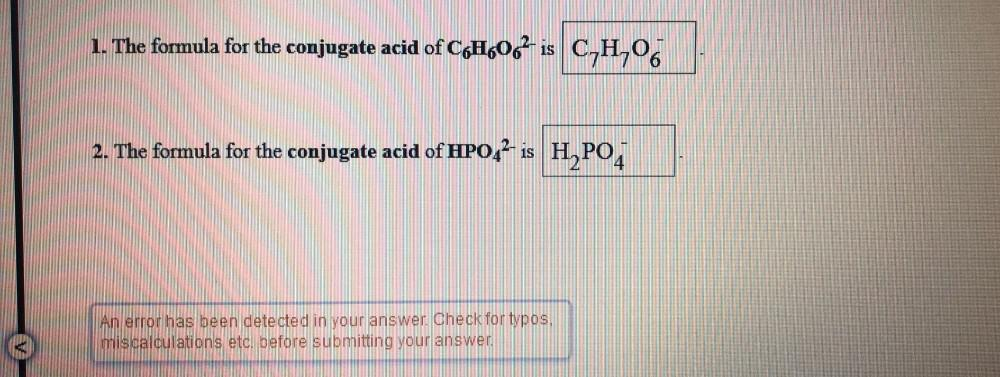Question:

# 1. The formula for the conjugate acid of C6H6O6^2- is ________ 2. The formula for the conjugate acid of HPO4^2- is __________1. The formula for the conjugate acid of C6H6O6^2- is ________ 2. The formula for the conjugate acid of HPO4^2- is __________